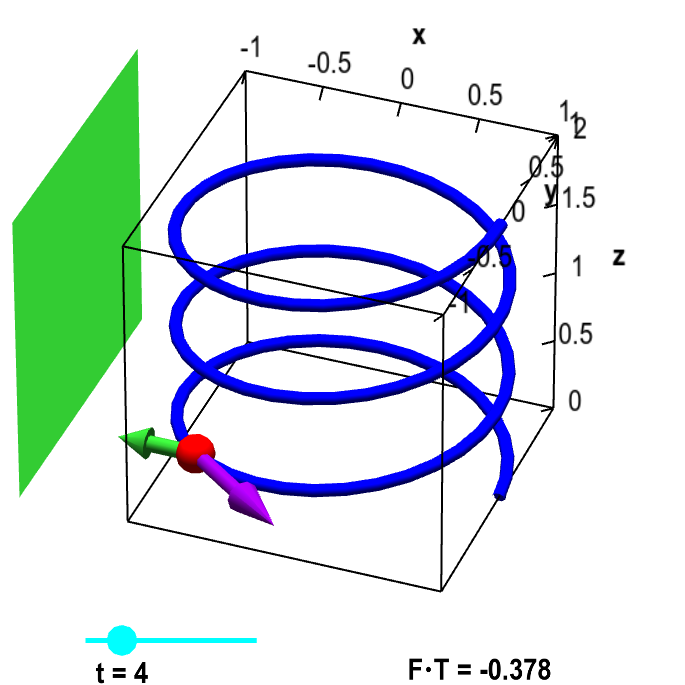# Math Insight

### Applet: Particle on helix with magnet and tangent vector#### Applet loading

The blue helix is parametrized by $\dllp(t) = (\cos t, \sin t, t/(3\pi))$, for $0 \le t \le 6\pi$. For a given value of $t$ (changed by the cyan point on the slider), the red point represents a magnetic bead at point $\dllp(t)$. The purple vector represents the tangent vector $\vc{T}$ in the direction of the particle's movement for increasing $t$. The green rectangle represents a large magnet, which induces the constant magnetic field represented by the vector field $\dlvf(x,y,z) = (-1/2, 0, 0)$ and illustrated with the green vector. The work done by the magnetic field on the particle is determined by the component $\dlvf \cdot \vc{T}$ of the magnetic field vector $\dlvf$ in the direction of the tangent vector $\vc{T}$, the value of which is shown in the lower right corner.

#### Applet links

This applet is found in the pages

List of all applets

#### Notation systems

More information on notation systems

#### General information about three.js applets

The applet was made using three.js and requires Javascript as well as a browser that supports WebGL. For most three.js applets, you can drag with the mouse to rotate the view, drag with the right button to pan, and zoom in/out with the mouse wheel. Many applets contain points that you can drag to change values of variables.